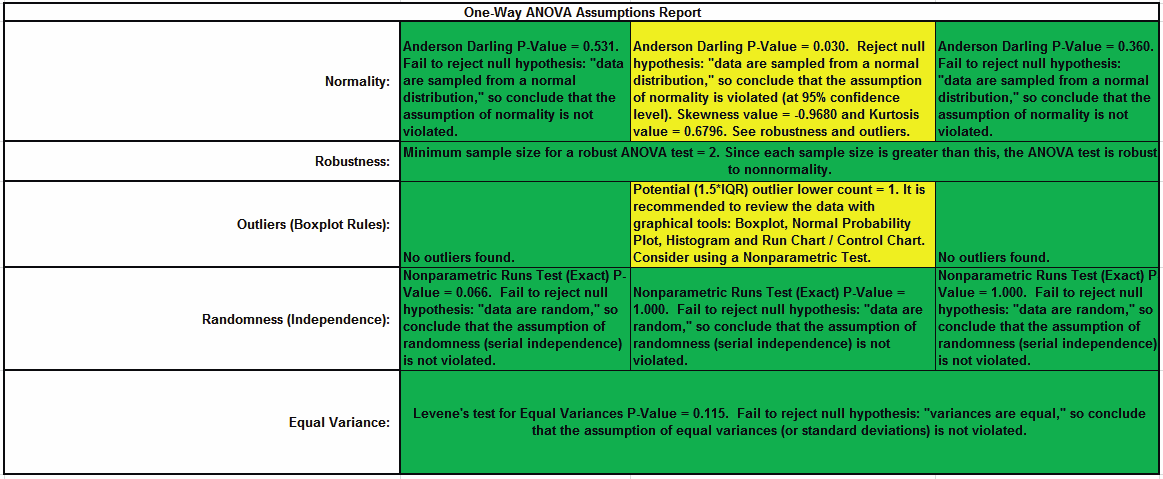Include Top

# How Do I Create a One-Way ANOVA & Means Matrix in Excel Using SigmaXL?

## One-Way ANOVA & Means Matrix

1. One-Way ANOVA and Means Matrix allows you to quickly do multiple pairwise comparisons. The One-Way ANOVA tests H0: μ1 = μ2 = μ3; Ha: at least one pairwise set of means are not equal.

2. Open Customer Data.xlsx, click on Sheet 1 tab.

3. Click SigmaXL > Statistical Tools > One-Way ANOVA & Means Matrix. Ensure that the entire data table is selected. If not, check Use Entire Data Table.

4. Click Next. Ensure that Stacked Column Format is checked. Select Overall Satisfaction, click Numeric Data Variable (Y) ; select Customer Type, click Group Category (X) . Uncheck Display ANOVA Table Details.Check Display Test Assumptions Report.5. Click OK. The results are shown below:

6.7. The ANOVA p-value of 0.0000 tells us that at least one pairwise set of means are not equal. From the Pairwise Mean Difference (Means Matrix), we conclude that Mean Overall Satisfaction is significantly different between Customer Type 2 and 3, as well as 1 and 2. Note that the default probabilities are Fisher Pairwise. The P-Values will be slightly different than the previous 2 Sample t-Test results because the variances from all 3 customer types are “pooled” here. This also results in slightly different confidence intervals. See below for more details on the multiple comparison options.

8. A graphical view of the Overall Satisfaction Mean and 95% Confidence Intervals are given to complement the Means Matrix. The fact that the CI’s for Customer Type 2 do not overlap those of Type 1 or 3, clearly shows that Customer Type 2 has a significantly higher mean satisfaction score. The overlap of CI’s for Type 1 and 3 shows that the mean satisfaction scores for 1 and 3 are not significantly different.

9. Note that the Confidence Level shown in the graph can be modified by clicking the Options button and setting Confidence Level. This also changes the alpha value used to highlight the PValues in red:

alpha = (100 – Confidence Level)/10

so the default Confidence Level = 95.0%, gives an alpha = 0.05

10. The R-Square (R-Sq) value of 20.95% indicates that Customer Type “explains” approximately 21% of the variation in Overall Satisfaction. We need to “drill down” to understand the root causes and best practices associated with Customer Type 2.

11. One-Way ANOVA Assumptions Report:This is a text report with color highlight: Green (OK), Yellow (Warning) and Red (Serious Violation).

Each sample is tested for Normality using the Anderson Darling test. If not normal, the minimum sample size for robustness of the ANOVA Test is determined utilizing Monte Carlo regression equations (see Basic Statistical Templates – Minimum Sample Size for Robust t-Tests and ANOVA). If the sample size is inadequate, a warning is given and the appropriate Nonparametric test is recommended (Kruskal-Wallis if there are no extreme outliers, Mood’s Median if there are extreme outliers).

Each sample is tested for Outliers defined as: Potential: Tukey's Boxplot (> Q3 + 1.5*IQR or < Q1 – 1.5*IQR); Likely: Tukey's Boxplot 2*IQR; Extreme: Tukey's Boxplot 3*IQR. If outliers are present, a warning is given and recommendation to review the data with a Boxplot and Normal Probability Plot. Here we have a potential outlier for Customer Type 2.

Tip: If the removal of outlier(s) result in an Anderson Darling P-Value that is > 0.1, a notice is given that excluding the outlier(s), the sample data are inherently normal.

Each sample is tested for Randomness using the Exact Nonparametric Runs Test. If the sample data is not random, a warning is given and recommendation to review the data with a Run Chart.

A test for Equal Variances is also applied. If all sample data are normal, Bartlett’s Test is utilized, otherwise Levene’s Test is used. If the variances are unequal, then a warning is given and Welch’s ANOVA is recommended

# Web Demos

Our CTO and Co-Founder, John Noguera, regularly hosts free Web Demos featuring SigmaXL and DiscoverSim